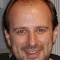# 0-1 Knapsack Iterative and Recursive with Code

## 0-1 Knapsack:

This problem can be solved be dynamic programming. Given some weight of items and their benefits / values / amount, we are to maximize the amount / benefit for given weight limit.

##### Background:

Suppose we are thief trying to steal. We got a knapsack with a weight carry limit. We go to a house there are a few items. The items have weights and also resale value. Now with our limited weight in the knapsack and many valuable items to take, we need to maximize our gain. We can do so by trying all items and filling the weight limit.

We will be given a few things as input. Such as number of items, each of their weights, each of their monetary value / cost ( if it represents cost ) and a weight limit. We are to find that by trying all items and the weight limit what is the maximum possible benefit.

If it is unclear the Wikipedia article on 0-1 knapsack may be helpful. I will show the table fill and items taken in the knapsack in another post.

##### Recurrence Relation:

The recurrence relation for this problem follows,

cost[ i, w ] = cost[ i – 1, w ] if, Wi > w
cost[ i, w ] = max( cost[ i – 1, w ], cost[ i – 1, w – W] ) if, Wi <= w

##### Question:
```items | weight | benefit
========================
1     | 6      | 10
2     | 1      | 5
3     | 2      | 7
4     | 5      | 12
5     | 4      | 8
6     | 3      | 6
```

For weight of 10 what is the maximum possible benefit by trying all item?

For this problem max benefit is 26.

The answer is always found in cost[ item_count, total_weight ]
Our item_count was 6 and total_weight was 10.
Here the answer is in cost[ 6, 10 ] which is 26.

## Code:

#### Iterative:

```/**
* @author  Quickgrid ( Asif Ahmed )
* Problem: Zero one knapsack iterative
*/

#include<stdio.h>

#define N 128

int CostTable[N][N];
int Weight[N];
int Benefit[N];

int total_weight = 10;
int item_count = 6;

inline int max(int a, int b){
return a > b ? a : b;
}

void dynamicKnapsack(){
int i = 0;

/*
* Set each column in first(zeroth) row to zero
*/
for(; i <= total_weight; ++i)
CostTable[i] = 0;

/*
* Set each row in first(zeroth) column to zero
*/
for(int i = 0; i <= item_count; ++i){
CostTable[i] = 0;

/*
* calculate till required weight
*/
for(int w = 1; w <= total_weight; ++w){
/*
* Get value from row above
* or,
* the value from a left column (w - Weight[i]) in the row above with added benefit
*/
if(Weight[i] <= w)
CostTable[i][w] = max(Benefit[i] + CostTable[i-1][w - Weight[i]], CostTable[i-1][w]);
else
CostTable[i][w] = CostTable[i-1][w];
}
}
}

void printCostTable(){
for(int i = 0; i <= item_count; ++i){
printf("%d:  ", i);
for(int w = 0; w <= total_weight; ++w)
printf("%d ", CostTable[i][w]);
printf("\n");
}
}

int main(){

Weight = 6;
Weight = 1;
Weight = 2;
Weight = 5;
Weight = 4;
Weight = 3;

Benefit = 10;
Benefit = 5;
Benefit = 7;
Benefit = 12;
Benefit = 8;
Benefit = 6;

dynamicKnapsack();

printf("Max Benefit: %d\n\n", CostTable[item_count][total_weight]);

printCostTable();

return 0;
}
```

#### Recursive:

```/**
* @author  Quickgrid ( Asif Ahmed )
* Problem: Zero one knapsack recursive
*/

#include<stdio.h>

#define N 128

int CostTable[N][N];
int Weight[N];
int Benefit[N];

int total_weight = 10;
int item_count = 6;

inline int max(int a, int b){
return a > b ? a : b;
}

int RecursiveKnapsack(int i, int w){
if(i == 0 || w == 0)
return 0;

if(Weight[i] > w)
return RecursiveKnapsack(i - 1, w);
else
return max(RecursiveKnapsack(i - 1, w), RecursiveKnapsack(i - 1, w - Weight[i]) + Benefit[i]);
}

int main(){

Weight = 6;
Weight = 1;
Weight = 2;
Weight = 5;
Weight = 4;
Weight = 3;

Benefit = 10;
Benefit = 5;
Benefit = 7;
Benefit = 12;
Benefit = 8;
Benefit = 6;

printf("Max Benefit: %d\n", RecursiveKnapsack(item_count, total_weight));

return 0;
}
```

#### Recursive Memoized:

```/**
* @author  Quickgrid ( Asif Ahmed )
* Problem: Zero one knapsack recursive memoization
*/

#include<stdio.h>

#define N 128

int CostTable[N][N];
int Weight[N];
int Benefit[N];

int total_weight = 10;
int item_count = 6;

inline int max(int a, int b){
return a > b ? a : b;
}

int RecursiveKnapsack(int i, int w){
if(CostTable[i][w] != -1)
return CostTable[i][w];

if(Weight[i] > w)
CostTable[i][w] = RecursiveKnapsack(i - 1, w);
else
CostTable[i][w] = max(RecursiveKnapsack(i - 1, w), RecursiveKnapsack(i - 1, w - Weight[i]) + Benefit[i]);
}

int main(){

Weight = 6;
Weight = 1;
Weight = 2;
Weight = 5;
Weight = 4;
Weight = 3;

Benefit = 10;
Benefit = 5;
Benefit = 7;
Benefit = 12;
Benefit = 8;
Benefit = 6;

/*
* Set all values of 2D matrix CostTable to Minus 1
*/
for(int i = 0; i <= item_count; ++i)
for(int w = 0; w <= total_weight; ++w)
CostTable[i][w] = -1;

printf("Max Benefit: %d\n", RecursiveKnapsack(item_count, total_weight));

return 0;
}
```

## 2 thoughts on “0-1 Knapsack Iterative and Recursive with Code”

1.saurabhk442 says:

Great article man. It helped me a lot. I had a doubt in the recursion. Shouldn’t it also contain the Benefit[i] when Wi<=w.

Like

2.Aris Pagourtzis says:

Thanks for the code. The Iterative method needs some slight modification though; Insert the following lines right after line 35 (otherwise it fills the first row with some numbers while it should be all zeroes):

}
for(int i = 1; i <= item_count; ++i){

Like